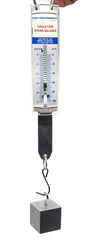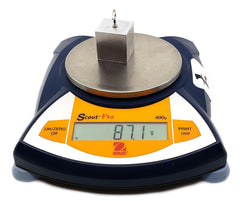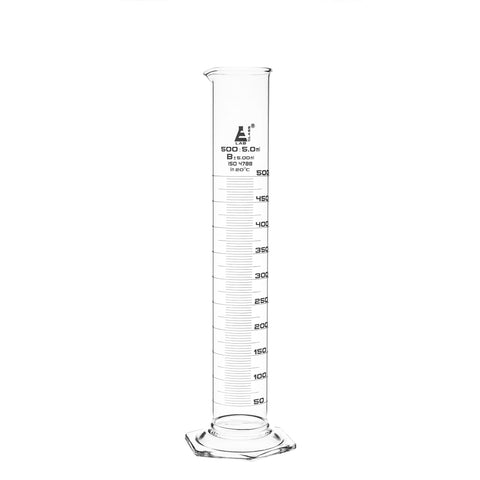# How to Measure the Density of an Unknown Material

Density is an inherent property of any material. Regardless of how much of it there is, or what shape it’s in, a material will always have the same density. Knowing the density of an item can help identify what something is made out of or where it came from.

The density of any material is determined by the ratio of its mass and volume. Represented mathematically:

d = m/V

Where d is the density, measured in grams per cubic centimeter (g/cm3), m is the mass, measured in grams (g), and V is the volume, measured in cubic centimeters (cm3). Another common way to measure density is in kilograms per cubic meter (kg/m3), which is simply 1000 times the same density at g/cm3.

To measure the density of an unknown material, you will need to find the mass and the volume. The mass can be found by using an electronic scale or spring balance.The volume can be measured by approximating the dimensions of an object, but a more reliable method is by using water displacement. Measure the volume of water poured into a graduated cylinder, then place the object in the water and remeasure the volume. The difference between the two volume measurements is the volume of the object.Now simply divide the mass by the volume to calculate the density of the object. You can compare this density to a table of common materials to see if it matches up with any of them.

Table of Common Material Density

 Material Density (g/cm3) Water (liquid) 1.00 Water (solid) 0.93 Aluminum 2.70 Iron 7.87 Brass 8.73 Copper 8.96 Gold 19.32

The density formula can be used with a known material to calculate mass or volume. If you have a chunk of iron that you want to find the weight of, you can measure the volume of the piece, then use the known density to find the mass.

m = d*V